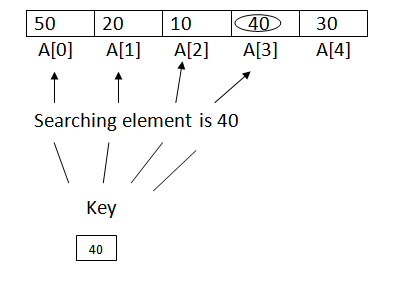# Linear search in Data Structures

21 September, 2018

Ratings

## Linear search

The linear search in data structures  or the sequential searching is most simple searching method. The key which is to be searched, is compared with each element of the list one by one in Linear Search If a match exits, the search is terminated in Linear Search If the end of the list is reached, it means that the search has failed and the key has no matching element in the list.   ex:### Program :

Linear search # include <stdio.h> # include <conio.h> int linear(int a),int n, int key); void main() { int a,n,i,key,p; clrscr(); printf(“Enter the value \n”); for (i=0; i<n; i++) { scanf(“%d”,&key); } printf (“Enter the search element \n”); scanf(“%d”,&key); p=liner(a,n,key); if(p>=0) { printf(“The element found at %d position”,p+1); } else printf(“The element is not found”); getch(); } int linear(int a, int n, int key) { int i=0; while(i<n) { if(a[i]==key) { return j; } i++; } return i; }

### Time complexity:

In the worst case(i.e when there)  N  comparisons are required hence the worst case performance represented as o(n); The best case in which the first comparison returns a match, it requires a single comparison and hence it is o(1) The average case roughly requires N/2 comparisons to search the element. That means the average time, as in worst-case is proportional to N and hence is o(n)